SLOS488F November   2006  – March 2015

PRODUCTION DATA.

1. Features
2. Applications
3. Description
4. Simplified Schematic
5. Revision History
6. Pin Configuration and Functions
7. Specifications
8. Detailed Description
1. 8.1 Overview
2. 8.2 Functional Block Diagram
3. 8.3 Feature Description
4. 8.4 Device Functional Modes
5. 8.5 Programming
6. 8.6 Register Maps
9. Applications and Implementation
1. 9.1 Application Information
2. 9.2 Typical Application
1. 9.2.1 Design Requirements
2. 9.2.2 Detailed Design Procedure
3. 9.2.3 Application Performance Curves
10. 10Power Supply Recommendations
11. 11Layout
12. 12Device and Documentation Support
13. 13Mechanical, Packaging, and Orderable Information

• RTJ|20
• YZH|16
• RTJ|20

## 7 Specifications

### 7.1 Absolute Maximum Ratings

over operating free-air temperature range (unless otherwise noted) (1)
MIN MAX UNIT
Supply voltage, VDD –0.3 6.0 V
VI Input voltage RIGHTINx, LEFTINx –2.5 3.6 V
SD, SCL, SDA –0.3 7 V
Output continuous total power dissipation See the Thermal Information table
TA Operating free-air temperature range –40 85 °C
TJ Operating junction temperature range –40 150 °C
Minimum Load Impedance 12.8 12.8 Ω
(1) Stresses beyond those listed under Absolute Maximum Ratings may cause permanent damage to the device. These are stress ratings only, which do not imply functional operation of the device at these or any other conditions beyond those indicated under Recommended Operating Conditions. Exposure to absolute-maximum-rated conditions for extended periods may affect device reliability.

### 7.2 Handling Ratings

MIN MAX UNIT
Tstg Storage temperature range –65 150 °C
V(ESD) Electrostatic discharge Human body model (HBM), per ANSI/ESDA/JEDEC JS-001, output pins(1) –8 8 kV
Human body model (HBM), per ANSI/ESDA/JEDEC JS-001, all other pins(1) –3.5 3.5 kV
Charged device model (CDM), per JEDEC specification JESD22-C101, all pins (2) –1500 1500 V
(1) JEDEC document JEP155 states that 500-V HBM allows safe manufacturing with a standard ESD control process.
(2) JEDEC document JEP157 states that 250-V CDM allows safe manufacturing with a standard ESD control process.

### 7.3 Recommended Operating Conditions

over operating free-air temperature range (unless otherwise noted)
MIN MAX UNIT
VDD Supply voltage 2.5 5.5 V
VIH High-level input voltage SCL, SDA, SD 1.3 V
VIL Low-level input voltage SCL, SDA 0.6 V
SD 0.35 V

### 7.4 Thermal Information

THERMAL METRIC(1) RTJ YZH UNIT
20 PINS 16 PINS
RθJA Junction-to-ambient thermal resistance 34.8 75 °C/W
RθJCtop Junction-to-case (top) thermal resistance 32.5 22
RθJB Junction-to-board thermal resistance 11.6 26
ψJT Junction-to-top characterization parameter 0.4 0.2
ψJB Junction-to-board characterization parameter 11.6 24
RθJCbot Junction-to-case (bottom) thermal resistance 3.1 N/A

### 7.5 Electrical Characteristics

TA = 25°C (unless otherwise noted)
PARAMETER TEST CONDITIONS MIN TYP MAX UNIT
|VOS| Output offset voltage VDD = 2.5 V to 5.5 V, inputs grounded 150 400 μV
PSRR Power supply rejection ratio VDD = 2.5 V to 5.5 V, inputs grounded –109 –90 dB
CMRR Common mode rejection ratio VDD = 2.5 V to 5.5 V –68 dB
|IIH| High-level input current VDD = 5.5 V, VI = VDD SCL, SDA 1 µA
SD 10
|IIL| Low-level input current VDD = 5.5 V, VI = 0 V SCL, SDA, SD 1 µA
IDD Supply current VDD = 2.5 V to 5.5 V, SD = VDD 4 6 mA
Shutdown mode, VDD = 2.5V to 5.5 V, SD = 0 V 0.4 1 µA
SW Shutdown mode, VDD = 2.5V to 5.5 V, SWS = 1 25 75 µA
Both HP amps disabled, VDD = 2.5V to 5.5 V,
SWS = 0, Charge Pump enabled, SD = VDD
1.4 2.5 mA

### 7.6 Operating Characteristics

VDD = 3.6 V , TA = 25°C, RL = 16 Ω (unless otherwise noted)
PARAMETER TEST CONDITIONS MIN TYP MAX UNIT
PO Output power Stereo, Outputs out of phase,
THD = 1%, f = 1 kHz,
Gain = 0.1 dB
VDD = 2.5V 60 mW
VDD = 3.6V 127
VDD = 5V 138
THD = 1%, f = 1 kHz,
Gain = 0.1 dB
VDD = 2.5V 110
VDD = 3.6V 230
VDD = 5V 290
THD+N Total harmonic distortion plus noise PO = 35 mW f = 100 Hz 0.0029%
f = 1 kHz 0.0055%
f = 20 kHz 0.0027%
kSVR Supply ripple rejection ratio 200 mVpp ripple, f = 217 Hz –97 –90 dB
200 mVpp ripple, f = 1 kHz –93
200 mVpp ripple, f = 20 kHz –76
ΔAv Gain matching 1%
Slew rate 0.3 V/µs
Vn Noise output voltage VDD = 3.6V, A-weighted, Gain = 0.1 dB 9 µVRMS
fosc Charge pump switching frequency 300 400 500 kHz
Start-up time from shutdown 5 ms
Differential input impedance See Figure 33
SNR Signal-to-noise ratio Po = 35 mW 98 dB
Thermal shutdown Threshold 180 °C
Hysteresis 35 °C
ZO Tri-state HP output impedance Hi-Z left and right bits set. HP amps disabled. DC value. 25
CO Output capacitance 80 pF

### 7.7 Timing Requirements(1)(2)

For I2C Interface Signals Over Recommended Operating Conditions (unless otherwise noted)
PARAMETER TEST CONDITIONS MIN TYP MAX UNIT
fSCL Frequency, SCL No wait states 400 kHz
tw(H) Pulse duration, SCL high 0.6 μs
tw(L) Pulse duration, SCL low 1.3 μs
tsu1 Setup time, SDA to SCL 300 ns
th1 Hold time, SCL to SDA 10 ns
t(buf) Bus free time between stop and start condition 1.3 μs
tsu2 Setup time, SCL to start condition 0.6 μs
th2 Hold time, start condition to SCL 0.6 μs
tsu3 Setup time, SCL to stop condition 0.6 μs
(1) VPull-up = VDD
(2) A pull-up resistor ≤2 kΩ is required for a 5 V I2C bus voltage.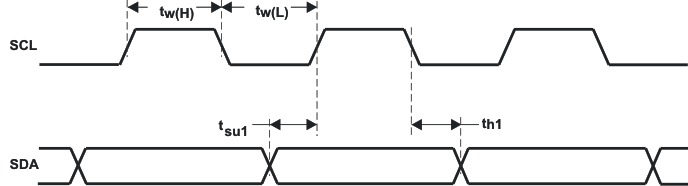Figure 1. SCL and SDA TimingFigure 2. Start and Stop Conditions Timing

### 7.8 Typical Characteristics

C(PUMP, DECOUPLE, ,BYPASS, CPVSS) = 1 μF, CI = 2.2µF.
All THD + N graphs taken with outputs out of phase (unless otherwise noted).

### Table 1. Table of Graphs

FIGURE
Total harmonic distortion + noise vs Output power Figure 3Figure 8
Total harmonic distortion + noise vs Frequency Figure 9Figure 22
Supply voltage rejection ratio vs Frequency Figure 23Figure 25
Common mode rejection ratio vs Frequency Figure 26, Figure 27
Output power vs Load Figure 28, Figure 29
Output voltage vs Load Figure 30, Figure 31
Power Dissipation vs Output power Figure 32
Differential Input Impedance vs Gain Figure 33
Shutdown time Figure 46
Startup time Figure 47RL = 16 Ω Gain = 6.1 dB fIN = 1 kHz BTL
Figure 3. Total Harmonic Distortion + Noise
vs Output PowerRL = 16 Ω Gain = 0.1 dB fIN = 1 kHz Stereo
Figure 5. Total Harmonic Distortion + Noise
vs Output PowerRL = 32 Ω Gain = 0.1 dB VDD = 3.6 fIN = 1 kHz Stereo
Figure 4. Total Harmonic Distortion + Noise
vs Output Power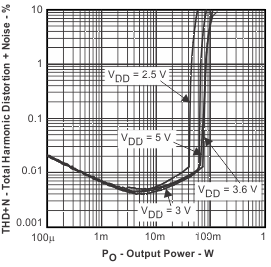RL = 32 Ω Gain = 0.1 dB fIN = 1 kHz Stereo
Figure 6. Total Harmonic Distortion + Noise
vs Output PowerRL = 16 Ω Gain = 6.1 dB fIN = 1 kHz BTL
Figure 7. Total Harmonic Distortion + Noise
vs Output PowerRL = 16 Ω VDD = 2.5 V Gain = 0.1 dB Stereo
Figure 9. Total Harmonic Distortion + Noise vs Frequency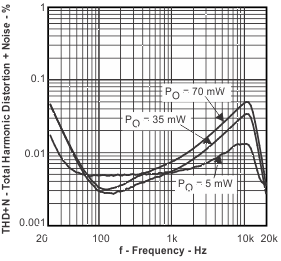RL = 16 Ω VDD = 3.6 V Gain = 0.1 dB Stereo
Figure 11. Total Harmonic Distortion + Noise vs FrequencyRL = 32 Ω VDD = 2.5 V Gain = 0.1 dB Stereo
Figure 13. Total Harmonic Distortion + Noise vs Frequency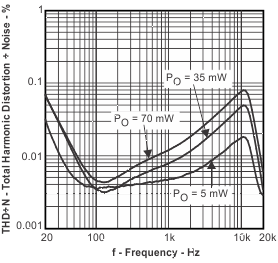RL = 32 Ω VDD = 3.6 V Gain = 0.1 dB Stereo
Figure 15. Total Harmonic Distortion + Noise vs FrequencyRL = 16 Ω VDD = 2.5 V Gain = 6.1 dB BTL
Figure 17. Total Harmonic Distortion + Noise vs FrequencyRL = 16 Ω VDD = 5 V Gain = 6.1 dB BTL
Figure 19. Total Harmonic Distortion + Noise vs FrequencyRL = 32 Ω VDD = 3.6 V Gain = 6.1 dB BTL
Figure 21. Total Harmonic Distortion + Noise vs FrequencyRL = 16 Ω Cp = 1 µF Gain = 0.1 dB Stereo
Figure 23. Supply Voltage Rejection Ratio vs FrequencyRL = 16 Ω Cp = 1 µF Gain = 6.1 dB BTL
Figure 25. Supply Voltage Rejection Ratio vs Frequency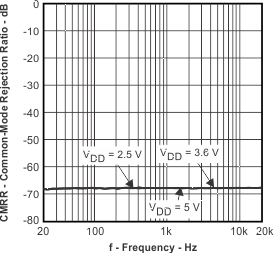RL = 16 Ω CI = 2.2 µF Gain = 6.1 dB BTL
Figure 27. Common Mode Rejection Ratio vs FrequencyfIN = 1 kHz Gain = 6.1 dB THD+N = 1% BTL
Figure 29. Output Power vs LoadfIN = 1 kHz Gain = 6.1 dB THD+N = 1% BTL
Figure 31. Output Voltage vs LoadVDD = 3.6 V
Figure 33. Differential Input Impedance vs GainRL = 32 Ω Gain = 6.1 dB fIN = 1 kHz BTL
Figure 8. Total Harmonic Distortion + Noise
vs Output PowerRL = 16 Ω VDD = 3 V Gain = 0.1 dB Stereo
Figure 10. Total Harmonic Distortion + Noise vs FrequencyRL = 16 Ω VDD = 5 V Gain = 0.1 dB Stereo
Figure 12. Total Harmonic Distortion + Noise vs FrequencyRL = 32 Ω VDD = 3 V Gain = 0.1 dB Stereo
Figure 14. Total Harmonic Distortion + Noise vs FrequencyRL = 32 Ω VDD = 5 V Gain = 0.1 dB Stereo
Figure 16. Total Harmonic Distortion + Noise vs Frequency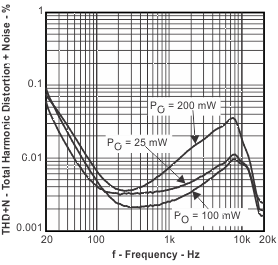RL = 16 Ω VDD = 3.6 V Gain = 6.1 dB BTL
Figure 18. Total Harmonic Distortion + Noise vs FrequencyRL = 32 Ω VDD = 2.5 V Gain = 6.1 dB BTL
Figure 20. Total Harmonic Distortion + Noise vs FrequencyRL = 32 Ω VDD = 5 V Gain = 6.1 dB BTL
Figure 22. Total Harmonic Distortion + Noise vs FrequencyRL = 32 Ω Cp = 1 µF Gain = 0.1 dB Stereo
Figure 24. Supply Voltage Rejection Ratio vs FrequencyRL = 16 Ω CI = 2.2 µF Gain = 0.1 dB Stereo
Figure 26. Common Mode Rejection Ratio vs Frequency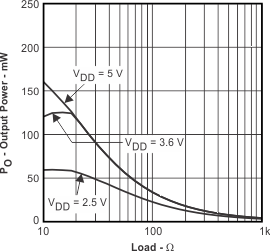fIN = 1 kHz Gain = 0.1 dB THD+N = 1% Stereo
Figure 28. Output Power vs LoadfIN = 1 kHz Gain = 0.1 dB THD+N = 1% Stereo
Figure 30. Output Voltage vs LoadRL = 16 Ω Gain = 0.1 dB Stereo
Figure 32. Power Dissipation vs Output Power